Chapter 5. Chemical Composition

# 5.1 Mass Terminology

### Learning Objectives

By the end of this section, you will be able to:

• Calculate formula masses for covalent and ionic compounds

We can argue that modern chemical science began when scientists started exploring the quantitative as well as the qualitative aspects of chemistry. For example, Dalton’s atomic theory was an attempt to explain the results of measurements that allowed him to calculate the relative masses of elements combined in various compounds. Understanding the relationship between the masses of atoms and the chemical formulas of compounds allows us to quantitatively describe the composition of substances.

## Formula Mass

In an earlier chapter, we described the development of the atomic mass unit, the concept of average atomic masses, and the use of chemical formulas to represent the elemental makeup of substances. These ideas can be extended to calculate the formula mass of a substance by summing the average atomic masses of all the atoms represented in the substance’s formula.

## Formula Mass for Covalent Substances

For covalent substances, the formula represents the numbers and types of atoms composing a single molecule of the substance; therefore, the formula mass may be correctly referred to as a molecular mass. Consider chloroform (CHCl3), a covalent compound once used as a surgical anesthetic and now primarily used in the production of the “anti-stick” polymer, Teflon. The molecular formula of chloroform indicates that a single molecule contains one carbon atom, one hydrogen atom, and three chlorine atoms. The average molecular mass of a chloroform molecule is therefore equal to the sum of the average atomic masses of these atoms. Figure 1 outlines the calculations used to derive the molecular mass of chloroform, which is 119.377 amu.

 1 C mass = 12.011 amu 1 H masses = 1.00794 amu 3 Cl masses = 3 x 35.4527 amu = 106.3581 Total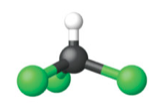= 119.377 amu = the molecular mass of CHCl3

Likewise, the molecular mass of an aspirin molecule, C9H8O4, is the sum of the atomic masses of nine carbon atoms, eight hydrogen atoms, and four oxygen atoms, which amounts to 180.15 amu (Figure 2).

 9 C mass = 9 x 12.011 amu = 108.099 amu 8 H masses = 8 x 1.00794 amu = 8.06352 amu 4 O masses = 4 x 15.9994 amu = 63.9976 amu Total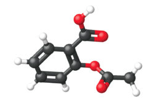= 180.160 amu = the molecular mass of C9H8O4

### Example 1

Ibuprofen, C13H18O2, is a covalent compound and the active ingredient in several popular nonprescription pain medications, such as Advil and Motrin. What is the molecular mass for this compound?

Solution
Molecules of this compound are comprised of 13 carbon atoms, 18 hydrogen atoms, and 2 oxygen atoms. Following the approach described above, the average molecular mass for this compound is therefore:

 13 C mass = 13 x 12.011 amu = 156.143 amu 18 H masses = 18 x 1.00794 amu = 18.14292 amu 2 O masses = 2 x 15.9994 amu = 31.9988 amu Total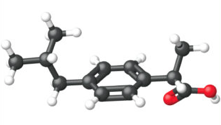= 206.285 amu = the molecular mass of C13H18O2

Test Yourself
Acetaminophen, C8H9NO2, is a covalent compound and the active ingredient in several popular nonprescription pain medications, such as Tylenol. What is the molecular mass for this compound?

151.16 amu

### Example 2

What is the molecular mass of each substance?

a) NBr3       b) C2H6

Solution

a) Add one atomic mass of nitrogen and three atomic masses of bromine:

 1 N mass = 14.0067 amu 3 Br masses = 3 × 79.904 amu = 239.712 amu Total = 253.719 amu = the molecular mass of NBr3

b) Add two atomic masses of carbon and six atomic masses of hydrogen:

 2 C masses = 2 × 12.011 amu = 24.022 amu 6 H masses = 6 × 1.00794 amu = 6.04764 amu Total = 30.070 amu = the molecular mass of C2H6

The compound C2H6 also has a common name—ethane.

Test Yourself

What is the molecular mass of each substance?

a) SO2         b) PF3

a) 64.065 amu         b) 87.969 amu

## Formula Mass for Ionic Compounds

Ionic compounds are composed of discrete cations and anions combined in ratios to yield electrically neutral bulk matter. The formula mass for an ionic compound is calculated in the same way as the formula mass for covalent compounds: by summing the average atomic masses of all the atoms in the compound’s formula. Keep in mind, however, that the formula for an ionic compound does not represent the composition of a discrete molecule, so it may not correctly be referred to as the “molecular mass.”

As an example, consider sodium chloride, NaCl, the chemical name for common table salt. Sodium chloride is an ionic compound composed of sodium cations, Na+, and chloride anions, Cl, combined in a 1:1 ratio. The formula mass for this compound is computed as 58.44 amu (Figure 3).

 1 Na mass = 22.9898 amu 1 Cl masses = 35.4527 amu Total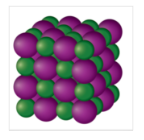= 58.4425 amu = the molecular mass of NaCl

Note that the average masses of neutral sodium and chlorine atoms were used in this computation, rather than the masses for sodium cations and chlorine anions. This approach is perfectly acceptable when computing the formula mass of an ionic compound. Even though a sodium cation has a slightly smaller mass than a sodium atom (since it is missing an electron), this difference will be offset by the fact that a chloride anion is slightly more massive than a chloride atom (due to the extra electron). Moreover, the mass of an electron is negligibly small with respect to the mass of a typical atom. Even when calculating the mass of an isolated ion, the missing or additional electrons can generally be ignored, since their contribution to the overall mass is negligible, reflected only in the nonsignificant digits that will be lost when the computed mass is properly rounded. The few exceptions to this guideline are very light ions derived from elements with precisely known atomic masses.

### Example 3

Aluminum sulfate, Al2(SO4)3, is an ionic compound that is used in the manufacture of paper and in various water purification processes. What is the formula mass (amu) of this compound?

Solution
The formula for this compound indicates it contains Al3+ and SO42− ions combined in a 2:3 ratio. For purposes of computing a formula mass, it is helpful to rewrite the formula in the simpler format, Al2S3O12. Following the approach outlined above, the formula mass for this compound is calculated as follows:

 2 Al mass = 2 x 26.9815 amu = 53.9630 amu 3 S masses = 3 x 32.066 amu = 96.198 amu 12 O masses = 12 x 15.9994 amu = 191.9928 amu Total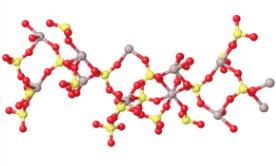= 342.154 amu = the molecular mass of Al2S3O12

Test Yourself
Calcium phosphate, Ca3(PO4)2, is an ionic compound and a common anti-caking agent added to food products. What is the formula mass (amu) of calcium phosphate?

310.18 amu

### Example 4

What is the molecular mass of Fe(NO3)3?

Solution

There is 1 atom of Fe, 3 atoms of N and 3(3) = 9 atoms of O

Thus, the molecular mass:

1(55.847amu) + 3(14.0067amu) + 9(15.9994amu) = 241.862 amu

Test Yourself

What is the mass, in amu’s, of 5.292 x 1021 molecules of Ni(NO3)2

9.669 x 1023 amu

### Chemistry Is Everywhere: Sulfur Hexafluoride

On March 20, 1995, the Japanese terrorist group Aum Shinrikyo (Sanskrit for “Supreme Truth”) released some sarin gas in the Tokyo subway system; twelve people were killed, and thousands were injured. Sarin (molecular formula C4H10FPO2) is a nerve toxin that was first synthesized in 1938 (Figure 4). It is regarded as one of the most deadly toxins known, estimated to be about 500 times more potent than cyanide. Scientists and engineers who study the spread of chemical weapons such as sarin (yes, there are such scientists) would like to have a less dangerous chemical, indeed one that is nontoxic, so they are not at risk themselves.

Sulfur hexafluoride is used as a model compound for sarin. SF6 (Figure 5) has a similar molecular mass (about 146 amu) as sarin (about 140 amu), so it has similar physical properties in the vapour phase. Sulfur hexafluoride is also very easy to accurately detect, even at low levels, and it is not a normal part of the atmosphere, so there is little potential for contamination from natural sources. Consequently, SF6 is also used as an aerial tracer for ventilation systems in buildings. It is nontoxic and very chemically inert, so workers do not have to take special precautions other than watching for asphyxiation.

Sulfur hexafluoride also has another interesting use: a spark suppressant in high-voltage electrical equipment. High-pressure SF6 gas is used in place of older oils that may have contaminants that are environmentally unfriendly (part (c) in the accompanying figure).

## Key Concepts and Summary

Isotopic mass (the mass of an isotope of an element, expressed in amu) of naturally occurring isotopes of a given element, is used to calculate the atomic mass of that element (expressed in amu).  The formula mass of a substance is the sum of the average atomic masses of each atom represented in the chemical formula and is expressed in atomic mass units. The formula mass of a covalent compound is also called the molecular mass.

### Exercises

1. a) What is the atomic mass of an oxygen atom?

b) What is the molecular mass of oxygen in its elemental form (meaning in the form it naturally occurs in)?

2. a) What is the atomic mass of bromine?

b) What is the molecular mass of bromine in its elemental form?

3. Determine the molecular mass of each substance.

a)  F2          b)  CO         c)  CO2

4.  Determine the mass of each substance.

a)  Na         b)  B2O3         c)  S2Cl2

5. Determine the formula mass of each substance.

a)  GeO2         b)  IF3         c)  XeF6

6. What is the total mass (amu) of carbon in each of the following molecules?

a) CH4         b) CHCl3         c) C12H10O6         d) CH3CH2CH2CH2CH3

7. Calculate the molecular or formula mass of each of the following:

a) P4         b) H2O         c) Ca(NO3)2         d) CH3CO2H (acetic acid)

e) C12H22O11 (sucrose, cane sugar)

8. Determine the molecular mass of the following compounds:

a)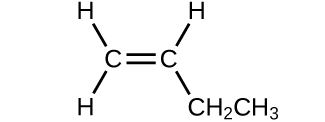b)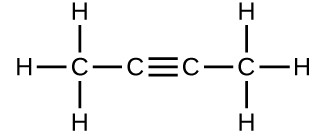c)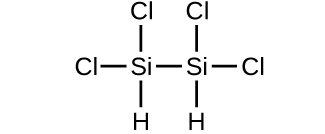d)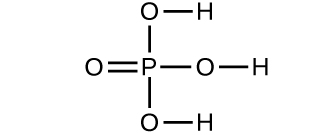9. Which molecule has a molecular mass of 28.05 amu?

a)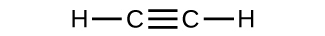b)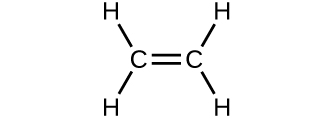c)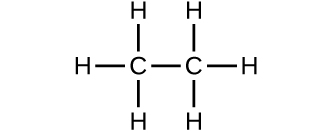1. a)  15.999 amu         b)  The elemental for of oxygen is O2.  Its moleculass mass is 31.998 amu.

2.  a) 79.904 amu         b) 159.808 amu

3. a)  37.997 amu         b)  28.010 amu         c)  44.010 amu

4. a)  22.9898 amu         b)  69.620 amu         c)  135.037 amu

5. a)  104.59 amu         b)  183.900 amu         c)  245.280 amu

6. a) 12.011 amu            b) 12.011 amu         c) 144.132 amu         d) 60.055 amu

7. a) 123.895 amu         b) 18.015 amu         c) 164.088 amu         d) 60.052 amu       e) 342.300 amu

8. a) 56.108 amu         b) 54.092 amu         c) 199.9977 amu         d) 97.9952 amu

9. B

## Glossary

formula mass: sum of the average masses for all atoms represented in a chemical formula; for covalent compounds, this is also the molecular mass